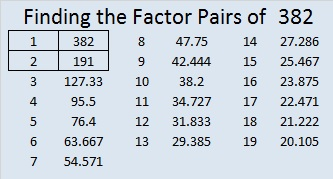# 382 and Level 5

382 is another even composite number. Scroll past the puzzle for its factoring information.Print the puzzles or type the factors on this excel file: 10 Factors 2015-02-02

• 382 is a composite number.
• Prime factorization: 382 = 2 x 191
• The exponents in the prime factorization are 1 and 1. Adding one to each and multiplying we get (1 + 1)(1 + 1) = 2 x 2 = 4. Therefore 382 has exactly 4 factors.
• Factors of 382: 1, 2, 191, 382
• Factor pairs: 382 = 1 x 382 or 2 x 191
• 382 has no square factors that allow its square root to be simplified. √382 ≈ 19.545382 is in only one Pythagorean triple: 382 – 36480 – 36482 which is 2 times (191 – 18240 – 18241).This site uses Akismet to reduce spam. Learn how your comment data is processed.